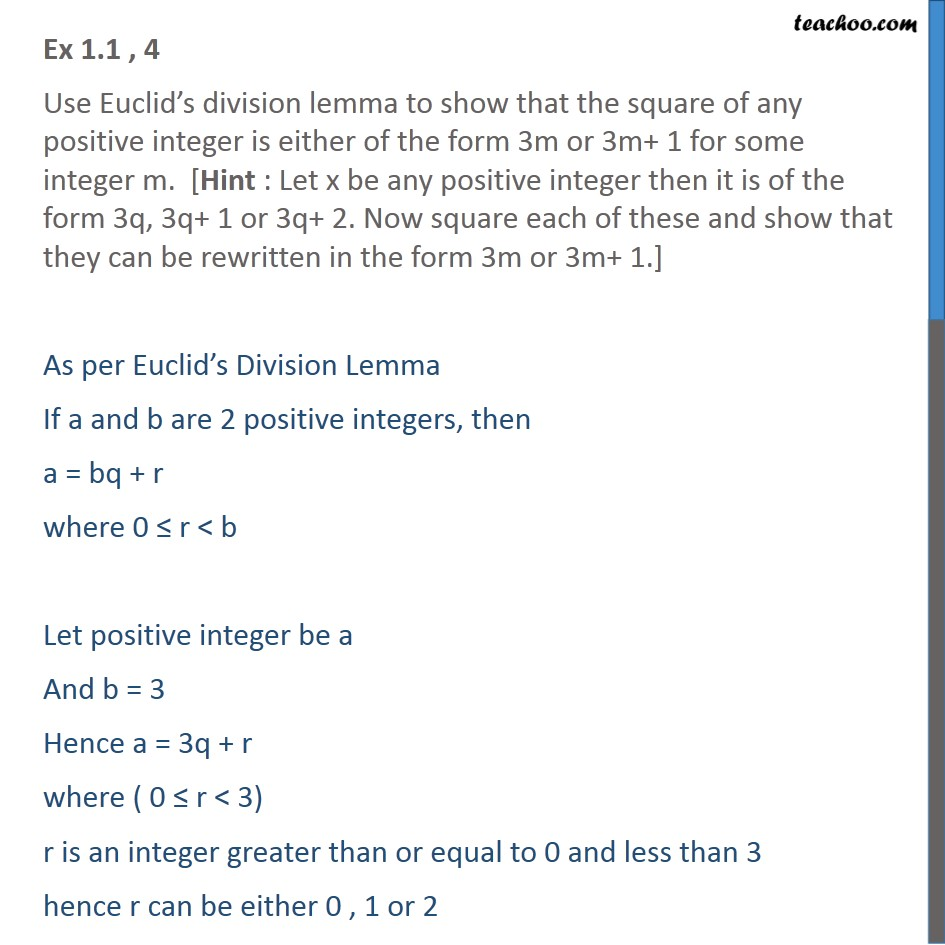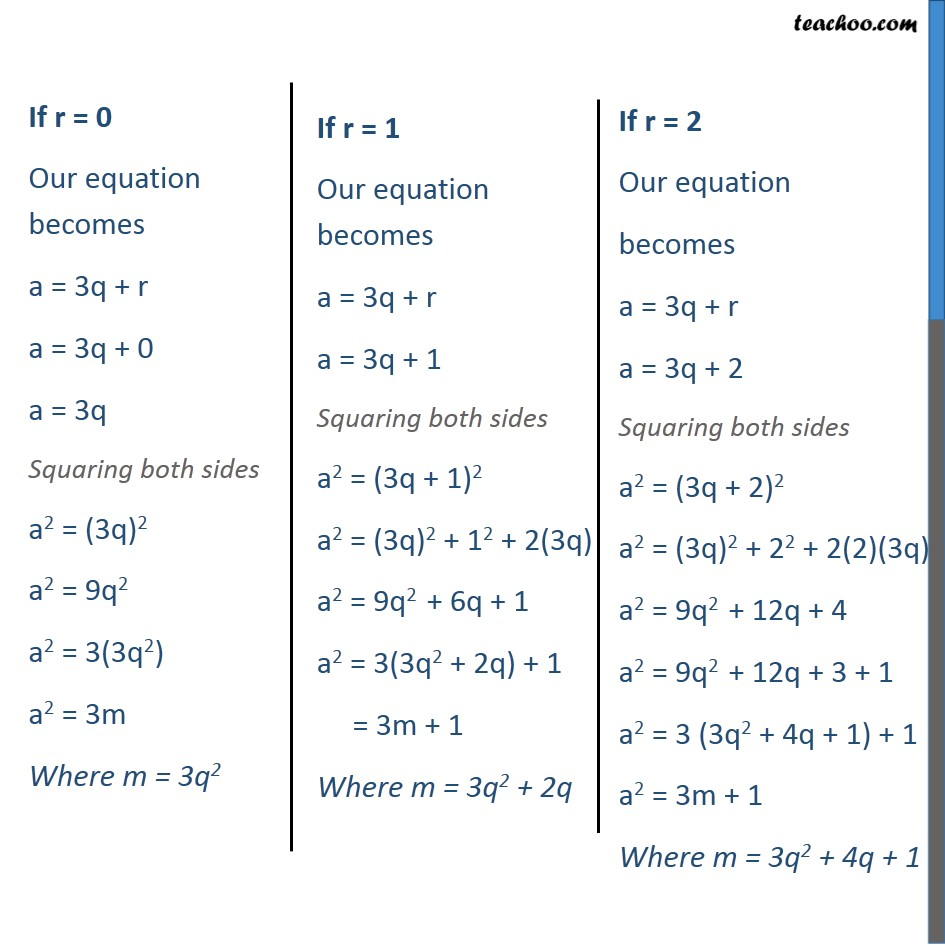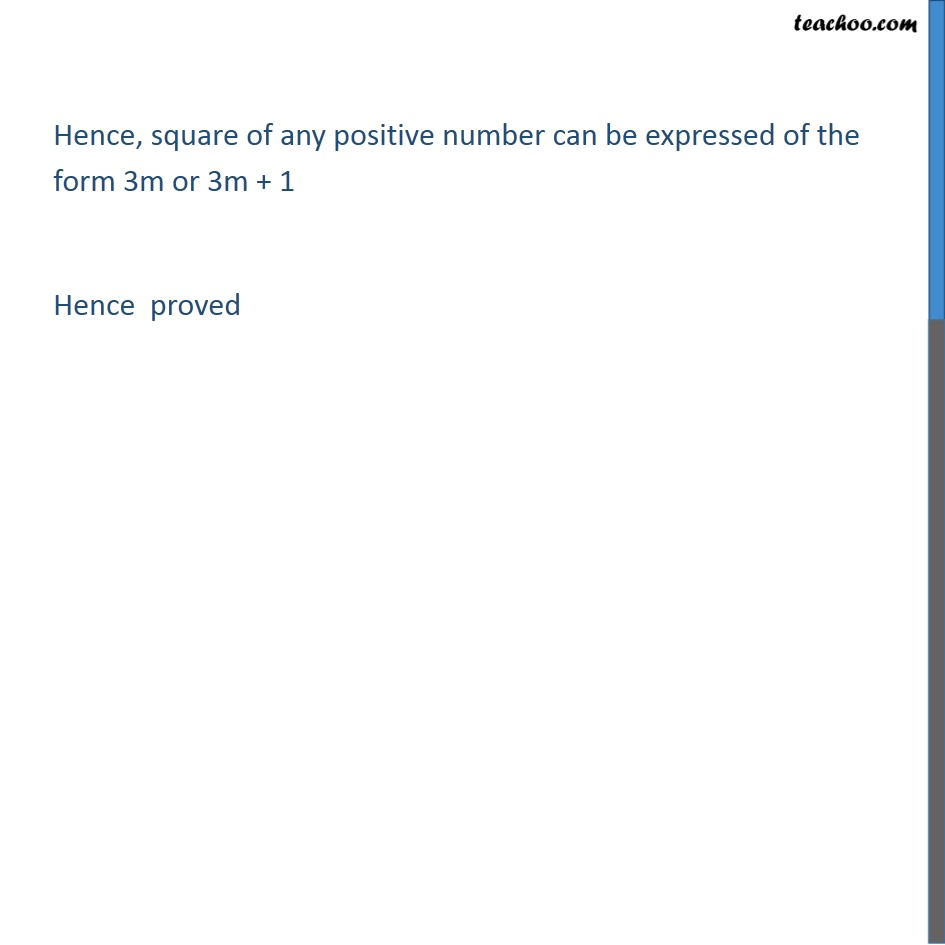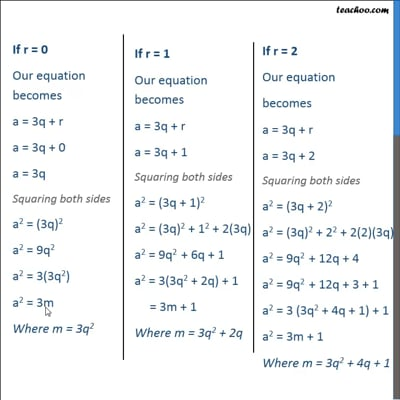Chapter 1 Class 10 Real Numbers

Class 10
Important Questions for Exam - Class 10This video is only available for Teachoo black users

Introducing your new favourite teacher - Teachoo Black, at only ₹83 per month

### Transcript

Ex 1.1 , 4 Use Euclid’s division lemma to show that the square of any positive integer is either of the form 3m or 3m+ 1 for some integer m. [Hint : Let x be any positive integer then it is of the form 3q, 3q+ 1 or 3q+ 2. Now square each of these and show that they can be rewritten in the form 3m or 3m+ 1.] As per Euclid’s Division Lemma If a and b are 2 positive integers, then a = bq + r where 0 ≤ r < b Let positive integer be a And b = 3 Hence a = 3q + r where ( 0 ≤ r < 3) r is an integer greater than or equal to 0 and less than 3 hence r can be either 0 , 1 or 2 Hence, square of any positive number can be expressed of the form 3m or 3m + 1 Hence proved